# Locally Coupled Oscillatory Recurrent Networks Learn Topographic Organization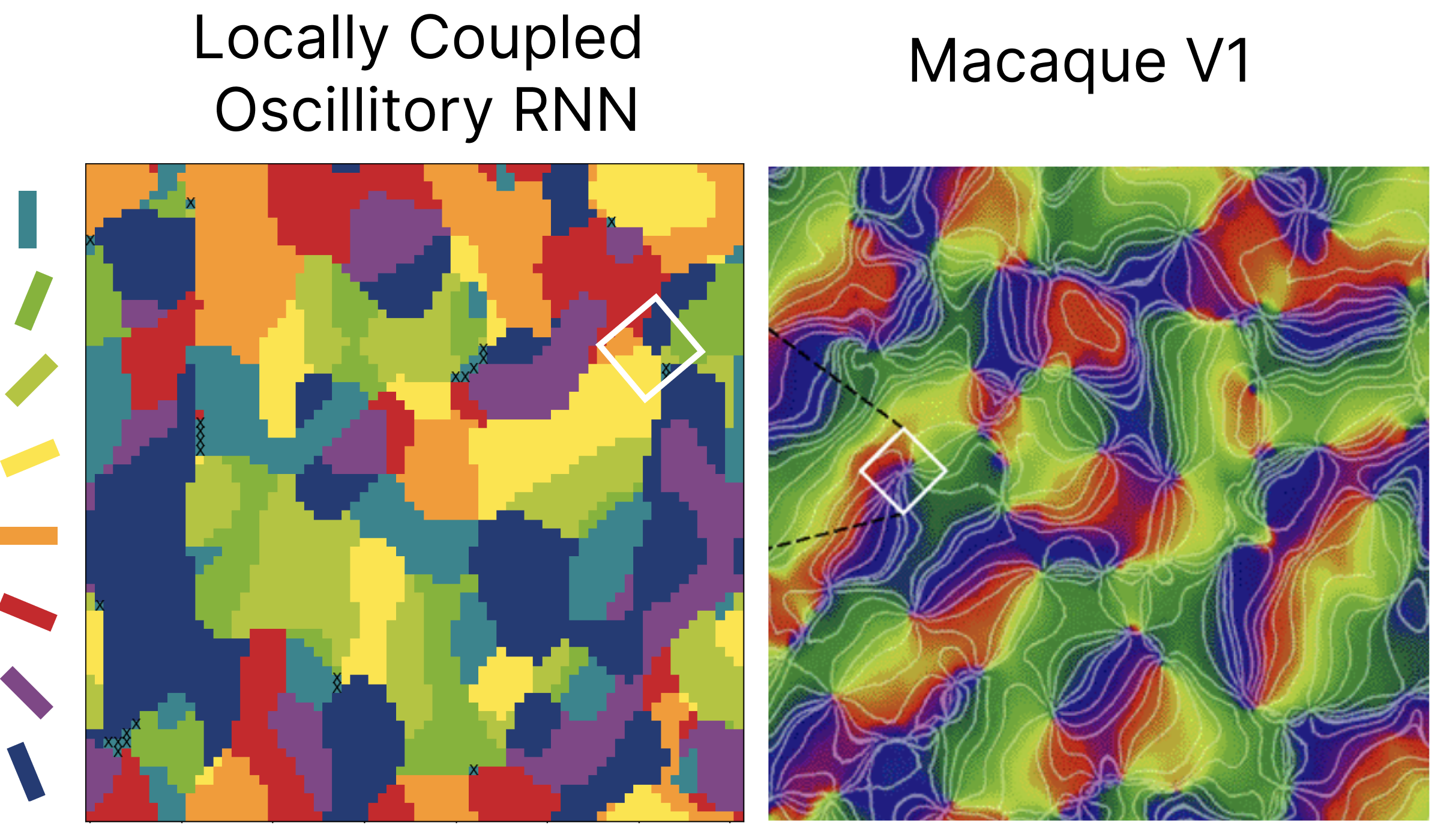Measured orientation selectivity of neurons, as color coded by the bars on the left. We see our LocoRNN’s simulated cortical sheet learns selectivity reminiscent of the orientation columns observed in the Macaque primary visual cortex (source: Principles of Neural Science. E. Kandel, J. Schwartz, T. Jessell, S. Siegelbaum, & A. Hudspeth. 2013.).

# Neural Wave Machines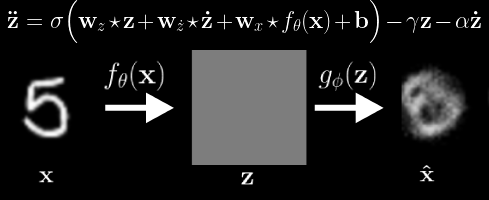Observed transformation (left), Latent Variable Waves (middle), and Reconstruction (right). We see the Neural Wave Machine learns to encode the observed transformations as traveling waves. In our paper, we show that such coordinated synchronous dynamics ultimately result in improved forecasting ability and efficiency when similarly modeling smooth continutous transformations as input.

# Homomorphic Self-Supervised Learning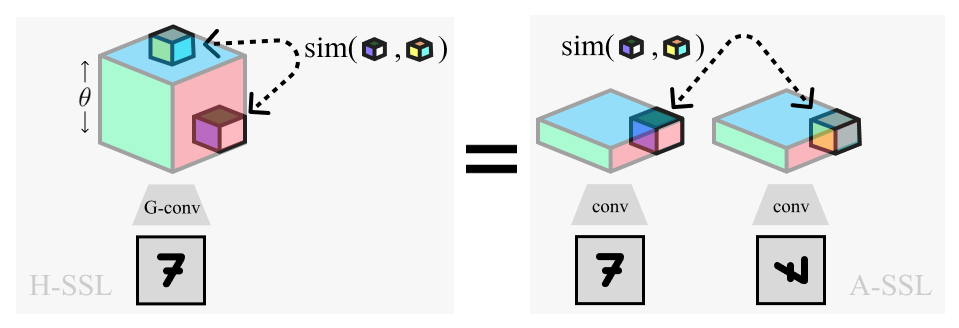Overview of Homomorphic-SSL (left) and its relation to traditional Augmentation-based SSL (right). Positive pairs extracted from the lifted dimension (θ) of a rotation equivariant network (G-conv) are equivalent to pairs extracted from the separate representations of two rotated images

# Modeling Category-Selective Cortical Regions with Topographic VAEs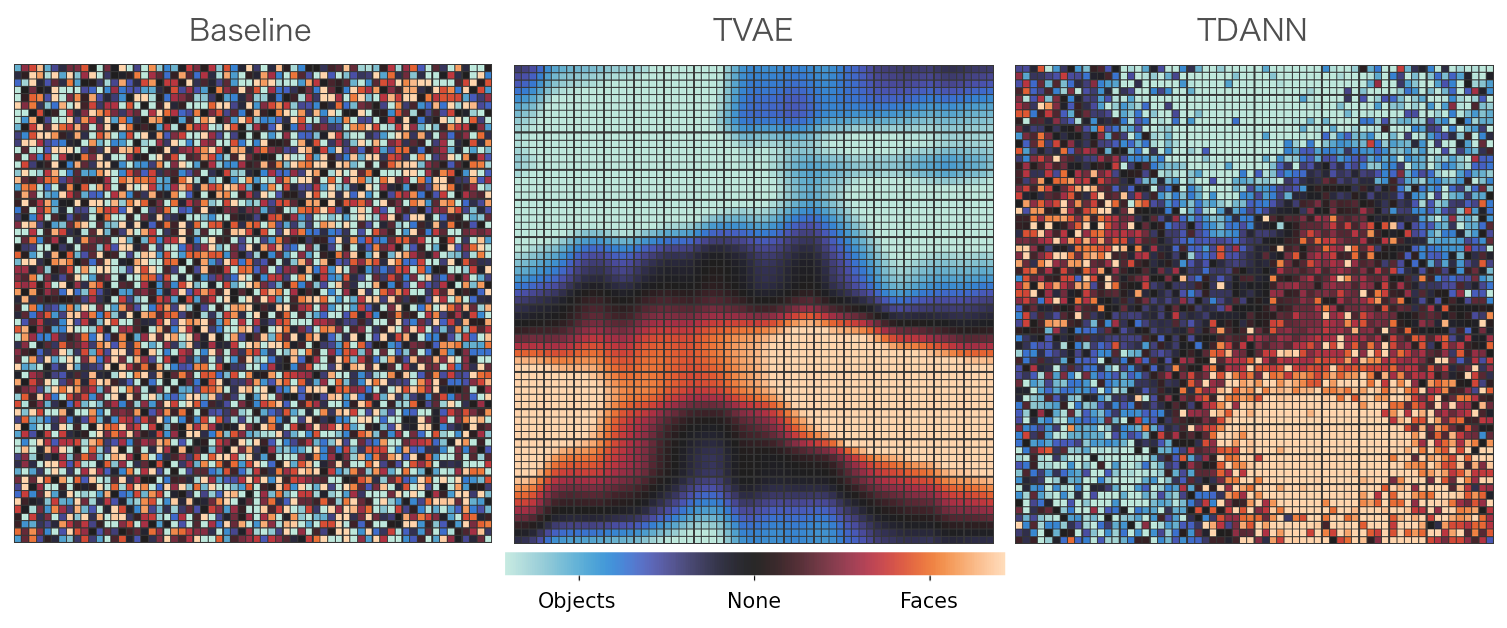Measurement of selectivity of top-layer neurons to images of Faces vs. images of Objects. The baseline pretrained Alexnet model (left) has randomly organized selectivity as expected. We see the Topographic VAE (middle) yeilds spatially dense clusters of neurons selective to images of faces, reminiscent of the ‘face patches’ observed in the primate cortex. The TVAE clusters are seen to be qualitatively similar to those produced by the supervised TDANN model of Lee et al. (2020) (right) without the need for class-labels during training.

# Predictive Coding with Topographic VAEs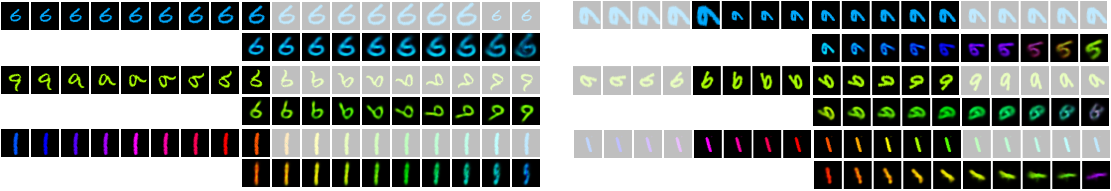We introduce a modification of the Topographic VAE, allowing it to be used in an online manner as a predictive model of the future. We observe that the Predictive Coding TVAE (PCTVAE) is able to learn more coherent sequence transformations (left) when compared with the original Topographic VAE (right).

# Topographic VAEs learn Equivariant Capsules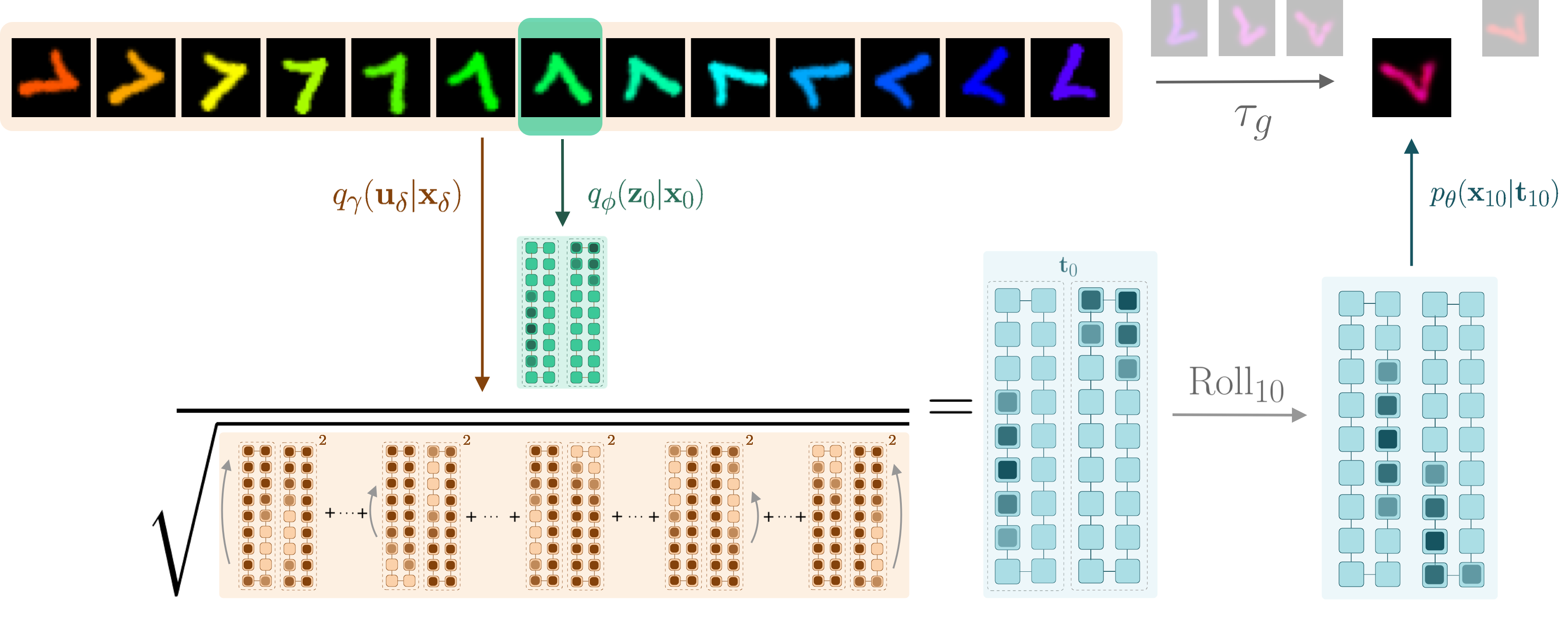Overview of the Topographic VAE with shifting temporal coherence. The combined color/rotation transformation in input space $$\tau_g$$ becomes encoded as a $$\mathrm{Roll}$$ within the equivariant capsule dimension. The model is thus able decode unseen sequence elements by encoding a partial sequence and rolling activations within the capsules. We see this completes a commutative diagram.

# As Easy as APC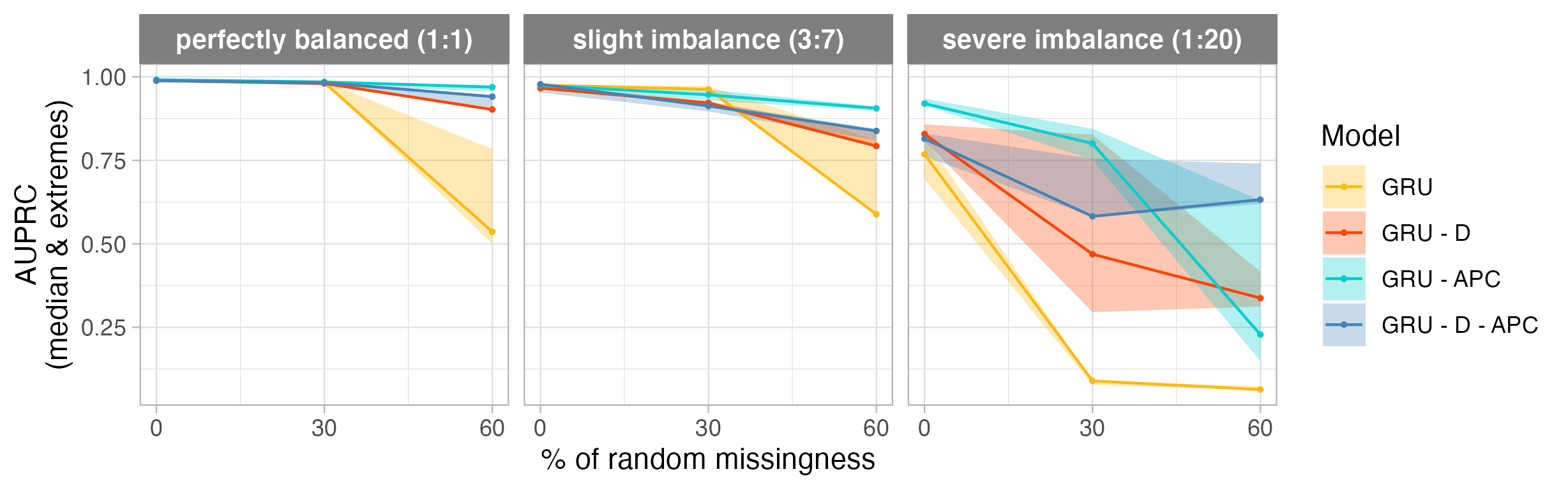Comparison of time series models as a function of missing data (x axis), and class imbalance (three plots). We see that baseline models (GRU & GRU-D with class-reweighting) perform significantly worse than models pre-trained with APC (auto-regressive predictive coding). These results suggest APC as an effective self-supervised learning method for handling missing data and class imbalance simultaneously.

# Self Normalizing Flows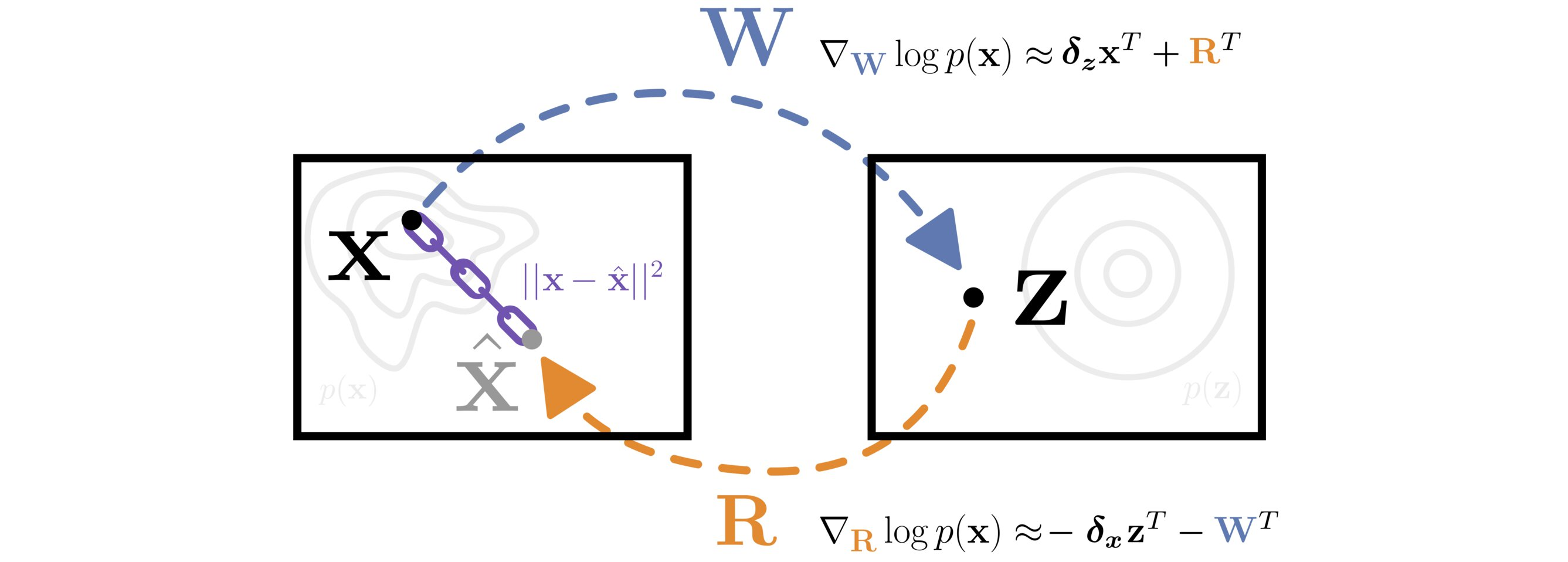A matrix $$\mathbf{W}$$ transforms data from $$\mathbf{X}$$ to $$\mathbf{Z}$$ space. The matrix $$\mathbf{R}$$ is constrained to approximate the inverse of $$\mathbf{W}$$ with a reconstruction loss $$||\mathbf{x} - \mathbf{\hat{x}}||^2$$. The likelihood of the data is efficiently optimized with respect to both $$\mathbf{W}$$ and $$\mathbf{R}$$ by approximating the gradient of the log Jacobian determinant with the learned inverse.

# Fast Weight Long Short-Term Memory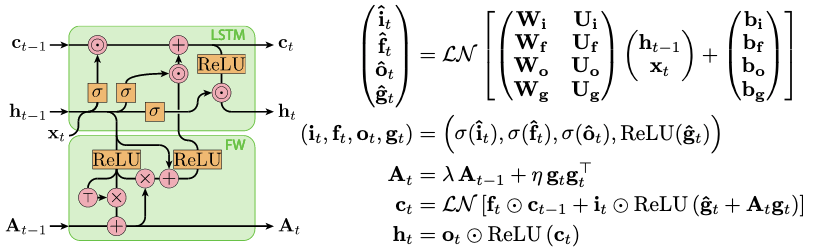A depiction of our gated Long Short-Term Memory cell augmented with an associative memory matrix A which is updated via the outer-product of the gated LSTM cell’s output.